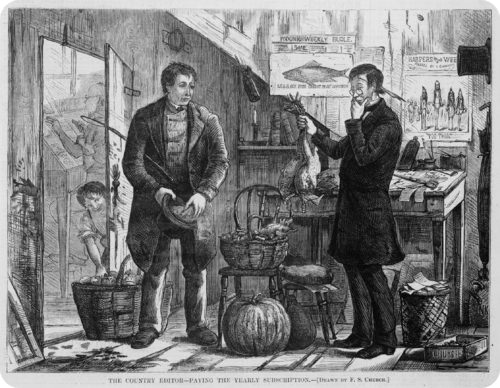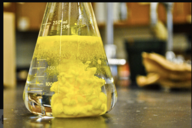# 11.9: Double Replacement Reactions

$$\newcommand{\vecs}{\overset { \rightharpoonup} {\mathbf{#1}} }$$ $$\newcommand{\vecd}{\overset{-\!-\!\rightharpoonup}{\vphantom{a}\smash {#1}}}$$$$\newcommand{\id}{\mathrm{id}}$$ $$\newcommand{\Span}{\mathrm{span}}$$ $$\newcommand{\kernel}{\mathrm{null}\,}$$ $$\newcommand{\range}{\mathrm{range}\,}$$ $$\newcommand{\RealPart}{\mathrm{Re}}$$ $$\newcommand{\ImaginaryPart}{\mathrm{Im}}$$ $$\newcommand{\Argument}{\mathrm{Arg}}$$ $$\newcommand{\norm}{\| #1 \|}$$ $$\newcommand{\inner}{\langle #1, #2 \rangle}$$ $$\newcommand{\Span}{\mathrm{span}}$$ $$\newcommand{\id}{\mathrm{id}}$$ $$\newcommand{\Span}{\mathrm{span}}$$ $$\newcommand{\kernel}{\mathrm{null}\,}$$ $$\newcommand{\range}{\mathrm{range}\,}$$ $$\newcommand{\RealPart}{\mathrm{Re}}$$ $$\newcommand{\ImaginaryPart}{\mathrm{Im}}$$ $$\newcommand{\Argument}{\mathrm{Arg}}$$ $$\newcommand{\norm}{\| #1 \|}$$ $$\newcommand{\inner}{\langle #1, #2 \rangle}$$ $$\newcommand{\Span}{\mathrm{span}}$$$$\newcommand{\AA}{\unicode[.8,0]{x212B}}$$Figure $$\PageIndex{1}$$ (Credit: F. S. Church, published in Harper's Weekly, January 17, 1874, p. 61; Source: http://commons.wikimedia.org/wiki/File:Barter-Chickens_for_Subscription.jpg(opens in new window); License: Public Domain)

The practice of barter (trading one thing for another) has been in existence for a very long time. In the illustration above, items like chickens were bartered for newspapers. You have something I want, and I have something you want. So we trade and we each have something new. Some chemical reactions are like that. Compounds swap parts and you have new materials.

## Double-Replacement Reactions

A double-replacement reaction is a reaction in which the positive and negative ions of two ionic compounds exchange places to form two new compounds. The general form of a double-replacement (also called double-displacement) reaction is:

$\ce{AB} + \ce{CD} \rightarrow \ce{AD} + \ce{CB}\nonumber$

In this reaction, $$\ce{A}$$ and $$\ce{C}$$ are positively-charged cations, while $$\ce{B}$$ and $$\ce{D}$$ are negatively-charged anions. Double-replacement reactions generally occur between substances in aqueous solution. In order for a reaction to occur, one of the products is usually a solid precipitate, a gas, or a molecular compound such as water.

### Formation of a Precipitate

A precipitate forms in a double-replacement reaction when the cations from one of the reactants combine with the anions from the other reactant to form an insoluble ionic compound. When aqueous solutions of potassium iodide and lead (II) nitrate are mixed, the following reaction occurs:

$2 \ce{KI} \left( aq \right) + \ce{Pb(NO_3)_2} \left( aq \right) \rightarrow 2 \ce{KNO_3} \left( aq \right) + \ce{PbI_2} \left( s \right)\nonumber$

There are very strong attractive forces that occur between $$\ce{Pb^{2+}}$$ and $$\ce{I^-}$$ ions and the result is a brilliant yellow precipitate (see figure below). The other product of the reaction, potassium nitrate, remains soluble.Figure $$\PageIndex{2}$$: Formation of lead iodide precipitate. (Credit: Paige Powers - "Lead Iodide"; Source: https://www.flickr.com/photos/44200742@N07/5533819494(opens in new window); License: CC-BY 2.0)

### Formation of a Gas

Some double-replacement reactions produce a gaseous product which then bubbles out of the solution and escapes into the air. When solutions of sodium sulfide and hydrochloric acid are mixed, the products of the reaction are aqueous sodium chloride and hydrogen sulfide gas.

$\ce{Na_2S} \left( aq \right) + 2 \ce{HCl} \left( aq \right) \rightarrow 2 \ce{NaCl} \left( aq \right) + \ce{H_2S} \left( g \right)\nonumber$

### Formation of a Molecular Compound

Another kind of double-replacement reaction is one that produces a molecular compound as one of its products. Many examples in this category are reactions that produce water. When aqueous hydrochloric acid is reacted with aqueous sodium hydroxide, the products are aqueous sodium chloride and water:

$\ce{HCl} \left( aq \right) + \ce{NaOH} \left( aq \right) \rightarrow \ce{NaCl} \left( aq \right) + \ce{H_2O} \left( l \right)\nonumber$

##### Example $$\PageIndex{1}$$: Double-Replacement Reactions

Write a complete and balanced chemical equation for the following double-replacement reactions. One product is indicated as a guide.

1. $$\ce{NaCN} \left( aq \right) + \ce{HBr} \left( aq \right) \rightarrow$$ (hydrogen cyanide gas is formed)
2. $$\ce{(NH_4)_2SO_4} \left( aq \right) + \ce{Ba(NO_3)_2} \left( aq \right) \rightarrow$$ (a precipitate of barium sulfate forms)
###### Solution

Step 1: Plan the problem.

In A, the production of a gas drives the reaction. In B, the production of a precipitate drives the reaction. In both cases, use the ionic charges of both reactants to construct the correct formulas of the products.

###### Step 2: Solve.

A. The cations of both reactants are $$+1$$ charged ions, while the anions are $$-1$$ charged ions. After exchanging partners, the balanced equation is:

$\ce{NaCN} \left( aq \right) + \ce{HBr} \left( aq \right) \rightarrow \ce{NaBr} \left( aq \right) + \ce{HCN} \left( g \right)\nonumber$

B. Ammonium ion and nitrate ion are $$1+$$ and $$1-$$ respectively, while barium and sulfate are $$2+$$ and $$2-$$. This must be taken into account when exchanging partners and writing the new formulas. Then, the equation is balanced.

$\ce{(NH_4)_2SO_4} \left( aq \right) + \ce{Ba(NO_3)_2} \left( aq \right) \rightarrow 2 \ce{NH_4NO_3} \left( aq \right) + \ce{BaSO_4} \left( s \right)\nonumber$

Both are double replacement reactions. All formulas are correct and the equations are balanced.

Occasionally, a reaction will produce both a gas and a molecular compound. The reaction of a sodium carbonate solution with hydrochloric acid produces aqueous sodium chloride, carbon dioxide gas, and water.

$\ce{Na_2CO_3} \left( aq \right) + 2 \ce{HCl} \left( aq \right) \rightarrow 2 \ce{NaCl} \left( aq \right) + \ce{CO_2} \left( g \right) + \ce{H_2O} \left( l \right)\nonumber$

## Summary

• The double-replacement reaction generally takes the form of AB + CDAD + CB where  A and C are positively-charged cations, while B and D are negatively-charged anions.
• In a double replacement reactions, typically one of the products is a precipitate, a gas, or a molecular compound.

## Review

1. In a double-replacement reaction, what type of compounds are usually the reactants? A double-replacement reaction occurs between sodium sulfide and hydrogen chloride. Write the rest of the chemical equation and balance it.
2. Na2S + HCl →
3. In double-replacement reaction, one of three possible types of products usually form. What are the three types?

This page titled 11.9: Double Replacement Reactions is shared under a CK-12 license and was authored, remixed, and/or curated by CK-12 Foundation via source content that was edited to the style and standards of the LibreTexts platform; a detailed edit history is available upon request.